# RD Sharma Solutions for Class 10 Maths Chapter 13 Probability Exercise 13.2

In this exercise, the concept of geometrical probability is tested with several interesting problems. For students, the RD Sharma Solutions Class 10 is a valuable resource to prepare for their qualifying exams. It contains detailed solutions to problems of all chapters as per the latest CBSE patterns. The RD Sharma Solutions for Class 10 Maths Chapter 13 Probability Exercise 13.2 PDF is provided below for further reference.

## RD Sharma Solutions for Class 10 Maths Chapter 13 Probability Exercise 13.2 Download PDF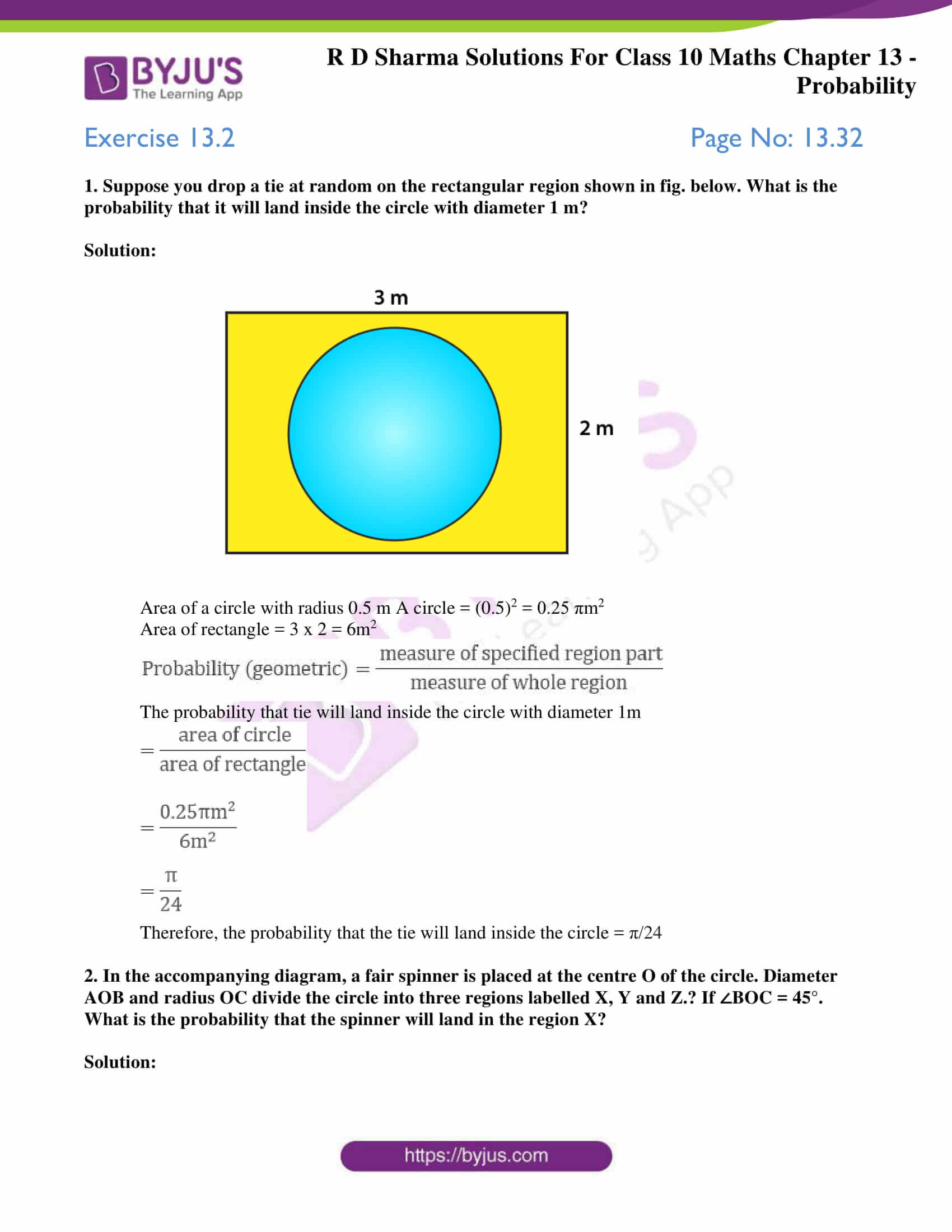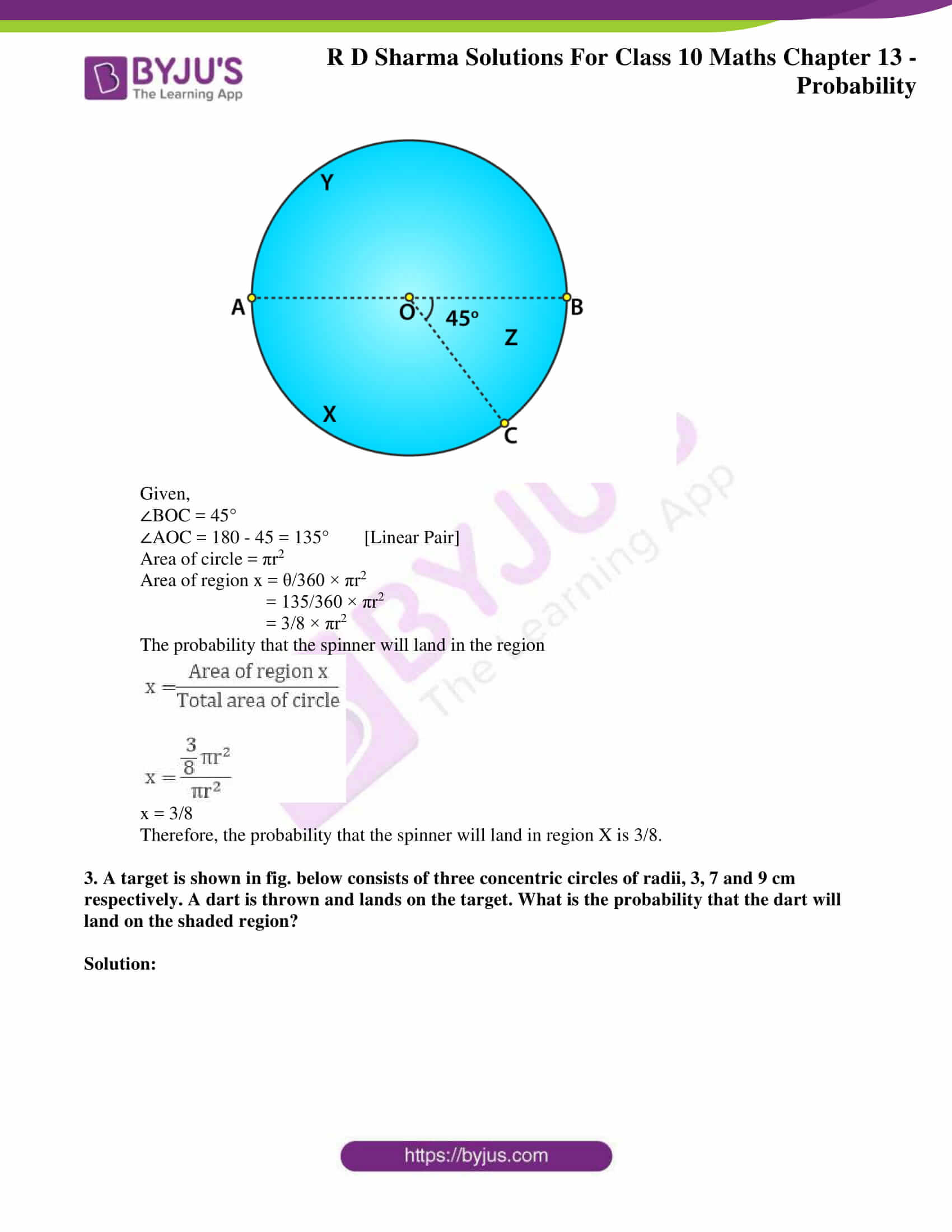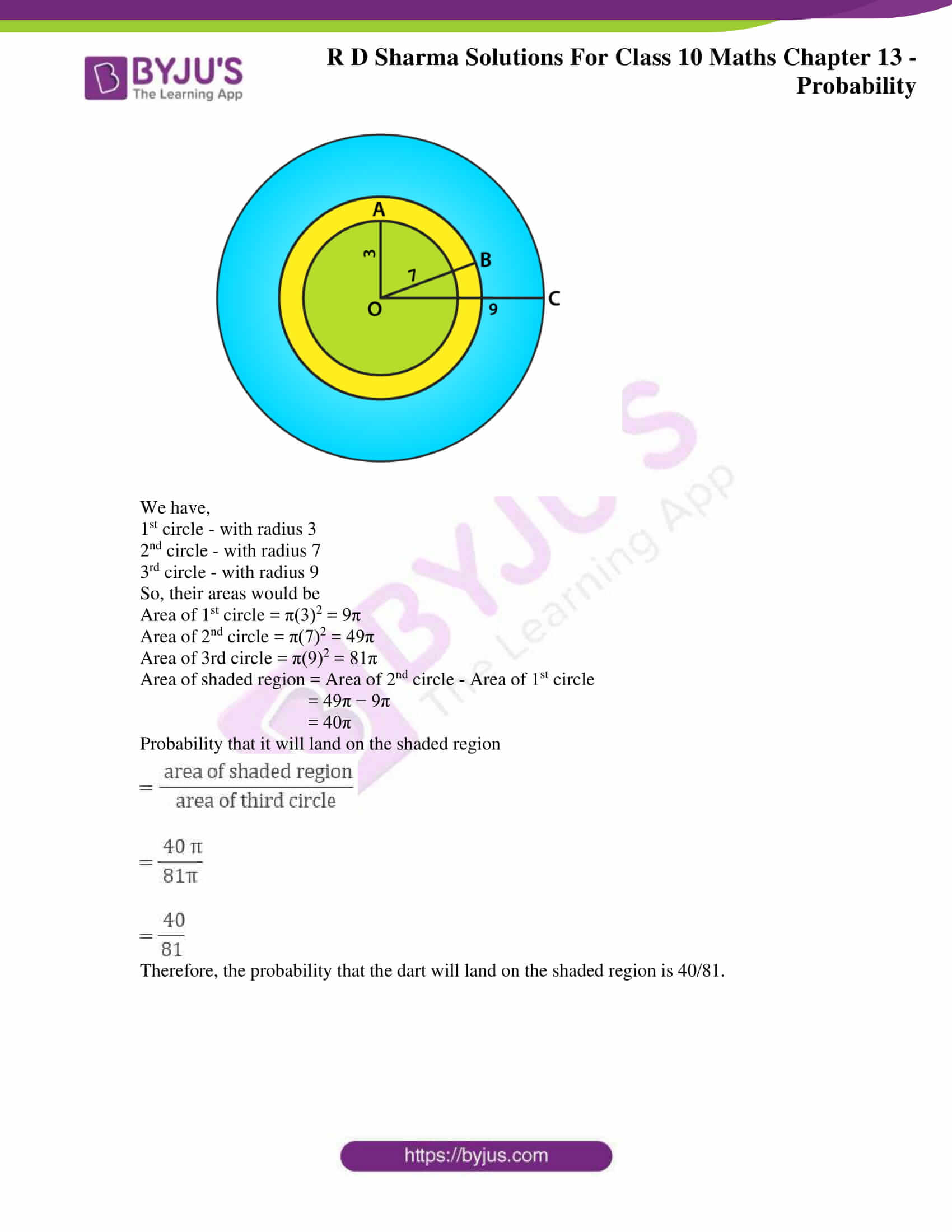### Access RD Sharma Solutions for Class 10 Maths Chapter 13 Probability Exercise 13.2

Exercise 13.2 Page No: 13.32

1. Suppose you drop a tie at random on the rectangular region shown in fig. below. What is the probability that it will land inside the circle with diameter 1 m?

Solution: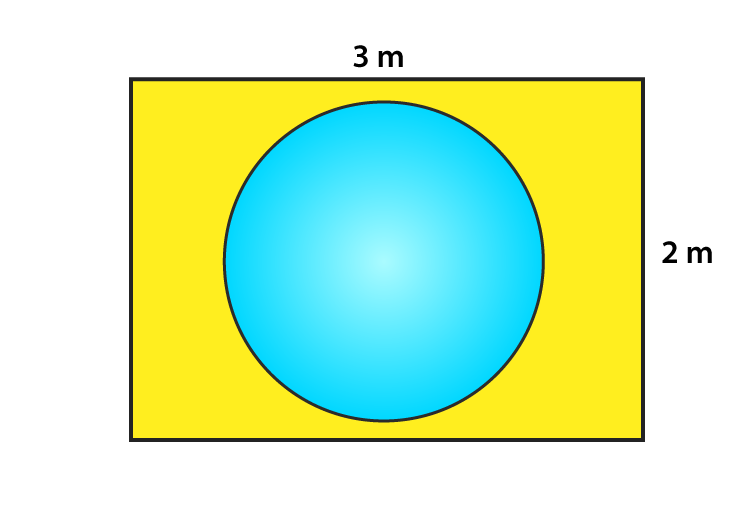Area of a circle with radius 0.5 m A circle = (0.5)2 = 0.25 πm2

Area of rectangle = 3 x 2 = 6m2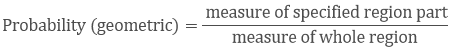The probability that tie will land inside the circle with diameter 1m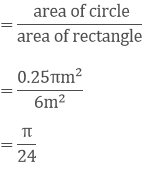Therefore, the probability that the tie will land inside the circle = π/24

2. In the accompanying diagram, a fair spinner is placed at the centre O of the circle. Diameter AOB and radius OC divide the circle into three regions labelled X, Y and Z.? If ∠BOC = 45°. What is the probability that the spinner will land in the region X?

Solution: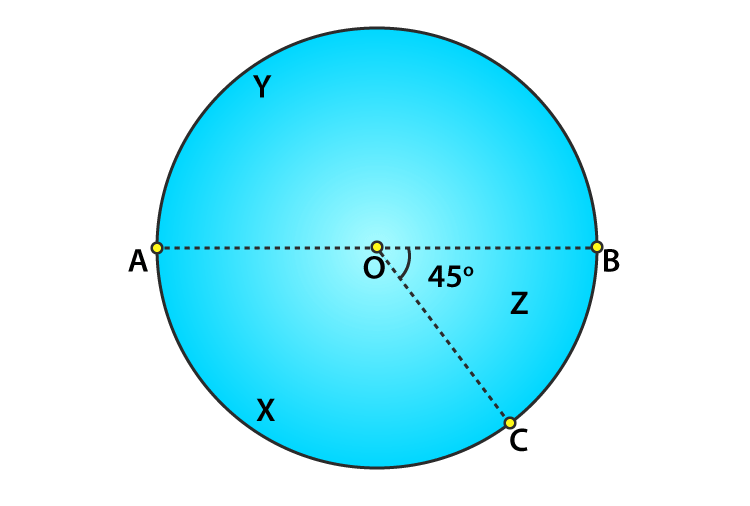Given,

∠BOC = 45°

∠AOC = 180 – 45 = 135° [Linear Pair]

Area of circle = πr2

Area of region x = θ/360 × πr2

= 135/360 × πr2

= 3/8 × πr2

The probability that the spinner will land in the region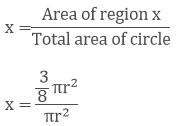x = 3/8

Therefore, the probability that the spinner will land in region X is 3/8.

3. A target is shown in fig. below consists of three concentric circles of radii, 3, 7 and 9 cm respectively. A dart is thrown and lands on the target. What is the probability that the dart will land on the shaded region?

Solution: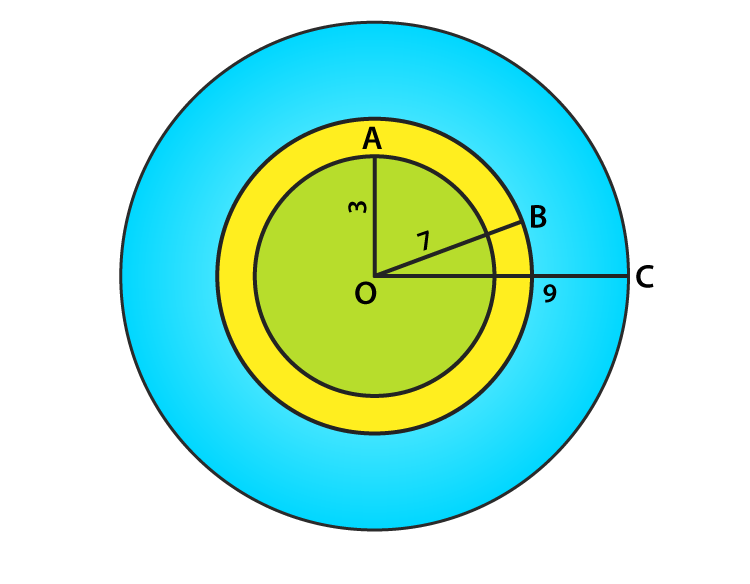We have,

1st circle – with radius 3

2nd circle – with radius 7

3rd circle – with radius 9

So, their areas would be

Area of 1st circle = π(3)2 = 9π

Area of 2nd circle = π(7)2 = 49π

Area of 3rd circle = π(9)2 = 81π

Area of shaded region = Area of 2nd circle – Area of 1st circle

= 49π − 9π

= 40π

Probability that it will land on the shaded region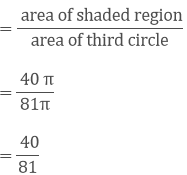Therefore, the probability that the dart will land on the shaded region is 40/81.